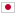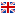Contest Duration: - (local time) (300 minutes) Back to Home
K - Permutation Period/Time Limit: 5 sec / Memory Limit: 512 MB

### Problem Statement

You have a permutation $p$ of $N$ integers. Initially $p_i = i$ holds for $1 \le i \le N$. For each $j \ (1 \le j \le N)$, let's denote $p^0_j = j$ and $p^k_j = p^{k-1}_{p_j}$ for any $k \ge 1$. The period of $p$ is defined as the minimum positive integer $k$ which satisfies $p^k_j = j$ for every $j \ (1 \le j \le N)$.

You are given $Q$ queries. The $i$-th query is characterized by two distinct indices $x_i$ and $y_i$. For each query, swap $p_{x_i}$ and $p_{y_i}$ and then calculate the period of updated $p$ modulo $10^9 + 7$ in the given order.

It can be proved that the period of $p$ always exists.

### Input

The input consists of a single test case of the following format.

$N \ Q$ $x_1 \ y_1$ $\vdots$ $x_Q \ y_Q$

The first line consists of two integers $N$ and $Q$ ($2 \le N \le 10^5, 1 \le Q \le 10^5$). The $(i+1)$-th line consists of two integers $x_i$ and $y_i$ ($1 \le x_i, y_i \le N, x_i \ne y_i$).

### Output

Print the answer in one line for each query.

### Sample Input 1

5 4
2 5
2 4
1 3
1 2


### Output for Sample Input 1

2
3
6
5


$p$ changes as follows: $[1,2,3,4,5] \to [1,5,3,4,2] \to [1,4,3,5,2] \to [3,4,1,5,2] \to [4,3,1,5,2]$.

### Sample Input 2

2 2
1 2
1 2


### Output for Sample Input 2

2
1


$p$ changes as follows: $[1,2] \to [2,1] \to [1,2]$.

### Sample Input 3

10 10
5 6
5 9
8 2
1 6
8 1
7 1
2 6
8 1
7 4
8 10


### Output for Sample Input 3

2
3
6
4
6
7
12
7
8
9